×
Get Full Access to Pitt - CHEM 0310 - Study Guide - Midterm
Get Full Access to Pitt - CHEM 0310 - Study Guide - Midterm

×

PITT / Chemistry / CHEM 0310 / What is the definition of a ring strain?

# What is the definition of a ring strain? Description

##### Description: This is a study guide made from my notes for the class. Everything that is in it may not be on the test but would still be good to know.
6 Pages 33 Views 2 Unlocks
Reviews

Study Guide Exam 1

## What is the definition of a ring strain?Red=Chapter Number Blue=Concept Green=Extra notes from Book

CHAPTER 1

∙ Coulombs Law

- Attracting force=constant*((+)charge*(-)charge))/distance^2 - a balance between the attractive forces and repulsions

-when the interaction is at its maximum, so is the stability while the  potential energy is at its minimum

∙ Kinds of Bonds

-covalent bonds: equally share valence electrons

-ionic bonds: one gives an electron and one takes an electron, separate charges

-polar covalent bond: between an ionic and covalent bond, have partial charges, give an electron but share it

-bonds determine the structure of a molecule by determining thee  reactions it can undergo

## What do you call the step by step process of a reaction?-making a bond releases energy while breaking a bond requires an  input of energy

The polarization of a bond is the consequence of a shift of the center of electron density in the bond toward the more electronegative atom. ∙ Octet Rule

-atoms will lose or gain electron to obtain a noble gas configuration -carbon can form 4 bonds because it needs 4 electrons

∙ Electronegativity

H=2.2 C=2.6 N=3.0 O=3.4 F=4.0 (most electronegative)

Cl=3.2 Br=3.0 I=2.7 B=1.9 Don't forget about the age old question of How to use protein sequencing to build a protein chain

-the longer the bonds are, the easier they are to break

-C-H bonds are very hard to break

∙ Kekule (Lewis) Structures

-first count the number of valence electrons in each atom, then bond  all atoms in your molecule together in the correct orientation (a bond is considered as two electrons), lastly complete octets with lone electron  pairs

## What is hyperconjugation?Important for predicting geometry and polarity.

∙ Resonance

-only structures with a double bond will go through resonance -resonance lowers the potential energy of the molecule and helps  stabalize it If you want to learn more check out How much the lip/mouth muscles are engaged in producing the sound?
If you want to learn more check out What were the developments in the 14th amendment?

Have characteristic property of being interconvertible by electron pair  movement only.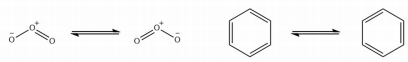-the true structure of the molecule is a composite of all the resonance  structures

-don’t tell about bond length or bond angle

∙ Orbitals

-particles can behave like a wave and we can think of electrons as  particle

-In phase interference is a bonding orbital and increases the  wavelenght We also discuss several other topics like How does social security run?

-Out of phase interference is an anti bonding orbital and it decrease  the wavelength

Quantum mechanics is the theory of electron motion.

-the two main orbitals we will work with are the s and p orbitals -s is symmetrical while p as asymmetrical and has three sub orbitals:  px, py, and pz

-Pauli exclusion principle: each orbital can only hold a maximum of two electrons

-Hunds rule: the second electron in an orbital must have the opposite  spin as the first

-Aufbau principle: must add an electron to each p orbital first before  adding a second electron

-the p orbitals are degenerate, they all have the same potential energy ∙ Bond Formation

-made by the inphase overlap of atomic orbitals

-the overlap is best between orbitals of similar size

-ther are two possibilities, the atoms bond or they do not bond ∙ Bond Order

-it is the number of bonding electrons minus the anitbonding electrons  all divided by 2. We also discuss several other topics like What are negative feedback loops?

-a sigma σ is a singel bond while π is involved in double and tripple  bonds

-π bonds are thee overlap of non-hybridized p orbitals, it is also a  perpendicular so it can not rotate freely

-σ can rotate freely and is symmetrical

∙ Hybridization

-is a product of mathematics

-often entails the mixinf or combining or orbitals

-sp3: made by mixing all 2p orbitals and the 2s orbital, creates a  tetrahedral shape with bond angles of 109 degrees, all σ bonds

-sp2: made by mixing two 2p orbitals with the 2s orbital, get trigonal  planar shape with 120 degree bonds, one σ bond and one π bond,  usually has a double bond We also discuss several other topics like What makes a cognized environment?

-sp: mad eby mixing one 2p orbital with the 2s orbital, get a linear  shape with 180 degree bond angles, one σ and two π bonds, usually a  triple bond

-remember that π bonds are weaker than σ bonds

∙ Isomers

-have the same molecular formula but differ in either structure or  conformations

-structural isomers have the same molecular formula and weightbut  have different structures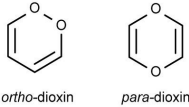CHAPTER 2

∙ Energetics/Kinematics

-thermodynamics {Energetics}, controls the extent of a reaction -kinetics, controls the speed of a reaction

Thermodynamic control yeilds the most stable products while kinetic  control is the product that forms the fastest.

-ΔG=ΔH-TΔS, usually negative for a spontaneous reaction -Rate= k[A] is a first order reaction

-Rate= k[A][B] is a second order reaction

∙ Acids/Bases

As a rule, the acidity of HA increase to the right and down in the  periodic table.

-pka=-logKa

-as the Ka increases, the acidity increase while the pka decreases -for electronegativity as it increases, the acidity increases and the pka  decreases

-as the s character in a hybridized bond increases, so doeas the acidity -Lewis acid: at least two electrons short of octet

-Lewis base: contains at least one lone electron pair

∙ Funtional Groups

-thegroup present in an organic molecule that imports reactivity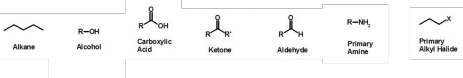∙ Alkanes (Hydrocarbons)

-general formula is CnH(2n+2)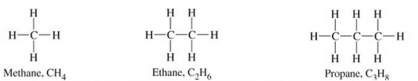∙ Conformations

-conformatns are isomers by rotatina bond

-if rotate, not changing electron density

-steric hindarance: causes the space between molecules/atoms, elctron repulsion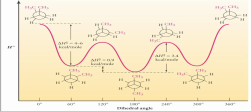CHAPTER 3

∙ Haloalkanes

-when a halogen bonds to an allkane group

-denoted as R-X

-heterolytic methane reaction creates either the carbocation or the  carbonanion by giving all of the electrons in one of the bonds between  carbon and hydrogen to one of the atoms

-CH3+ or CH3-

-in a homolytic reaction, each atom recevies one electron from the  bond, creates the methane radical

-the DH or dissociation energy is a quantitative measure of the bond  strength

-if the DH increases then so to does the bond strength

∙ Hyperconjugation

-the delocalization of the one electron in a radical

-reduces the potential energy

-the number of hyperconjugations increases as the number of CH3 in a  molecule increases

-ΔH= Ebreak-Eformation

∙ Mechanism

-the step by step process of a reaction

Here are the steps for a chlorination reaction:

-Initiation: Cl-Cl → ∙Cl + ∙Cl

-Propagation: ∙Cl + H-CH3 → H-Cl + ∙CH3

Cl-Cl + ∙CH3 → CH3-Cl + ∙Cl

-Termination: ∙Cl + ∙Cl → Cl-Cl

OR ∙CH3 + ∙Cl → CH3-Cl

OR ∙CH3 + ∙CH3 → CH3-CH3

-the radical is less stable so it as a higher energy transition state -early and late transition state: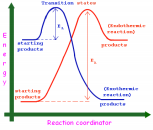-the selectivity ratio for chlorine is 1:4:5 for if the chlorine bonds to a  primary carbon, a secondary carbon, or a tertiary carbon. -we only know the selectivetiy ratio between a primary and a tertiary  carbon for flourine and bromine and the ratios are 1:1.5 and 1:1000  respectively

-the most selective molecule is bromine and the least selective is  chlorine, we don’t even mention iodine because it is not a spontaneous  reaction

-as the reactivity of a halide increases then the selectivity decreases

CHAPTER 4

∙ Cycloalkanes

-cyclopropane:

-cyclobutane:

-cyclopentane:

-cyclohexane:

-the general formula for cycloalkanes is CnH2n

-trans and cis are not conformational isomers but stereoisomers, thay  have different spatial arrangements

-the average change in energy when you get rid of a CH2(methylene) is 157.4 kcal/mol

∙ Ring Strain

-it is the angle strain plus the eclipsing strain

-cyclopropane has the highest ring strain while cyclohexane has the  least ring strain

-the bond angles in cylcopropane, cyclobutane, and cyclopentane are  not 109⁰ and so this creates strain in the sp3 hybridization angles -cyclopropane has all of its hydrogens eclipsing

-cyclobutane has a partial standard structure, due to its extra CH2 group it has more flexibility

-cyclohexane has two conformations: chair and boat

-the chair conformation for cyclohexane is the most stable  conformation when constituents are in the equatorial position and not  the axial(ꓕ)

-axial hydrogens or constituents are involved in steric hindarance

Page ExpiredIt looks like your free minutes have expired! Lucky for you we have all the content you need, just sign up here